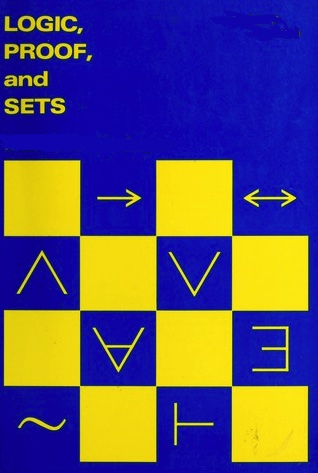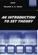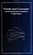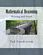Processing ......FreeComputerBooks.com Links to Free Computer, Mathematics, Technical Books all over the World

Proof, Sets, and Logic
Top Free Algorithms Books 🌠 - 100% Free or Open Source!
• Title Proof, Sets, and Logic
• Author(s): M. Randall Holmes
• Publisher: Boise State University (5/15/2023)
• Paperback: N/A
• eBook: PDF (475 pages)
• Language: English
• ISBN-10: N/A
• ISBN-13: N/ABook Description

Addressing the importance of constructing and understanding mathematical proofs, this book introduces key concepts from logic and set theory as well as the fundamental definitions of algebra to prepare readers for further study in the field of mathematics. The author supplies a seamless, hands-on presentation of number systems, utilizing key elements of logic and set theory and encouraging readers to abide by the fundamental rule that you are not allowed to use any results that you have not proved yet.

The book begins with a focus on the elements of logic used in everyday mathematical language, exposing readers to standard proof methods and Russell's Paradox. Once this foundation is established, subsequent chapters explore more rigorous mathematical exposition that outlines the requisite elements of Zermelo-Fraenkel set theory and constructs the natural numbers and integers as well as rational, real, and complex numbers in a rigorous, yet accessible manner.

Abstraction is introduced as a tool, and special focus is dedicated to concrete, accessible applications, such as public key encryption, that are made possible by abstract ideas.

The book concludes with a self-contained proof of Abel's Theorem and an investigation of deeper set theory by introducing the Axiom of Choice, ordinal numbers, and cardinal numbers.

This is an excellent book for mathematics courses on proofs, logic, and set theory at the upper-undergraduate level as well as a supplement for transition courses that prepare students for the rigorous mathematical reasoning of advanced calculus, real analysis, and modern algebra. The book is also a suitable reference for professionals in all areas of mathematics education who are interested in mathematical proofs and the foundation upon which all mathematics is built.

Reviews, Ratings, and Recommdations: Related Book Categories: Read and Download Links:Similar Books:
•Language, Proof and Logic (Jon Barwise, et al)

This book covers first-order language in a method appropriate for first and second courses in logic, and is specially useful to undergraduates of philosophy, computer science, mathematics, and linguistics.

•An Introduction to Set Theory (William A. R. Weiss)

This book covers the basics: relations, functions, orderings, finite, countable, and uncountable sets, and cardinal and ordinal numbers, gives students sufficient grounding in a rigorous approach to the revolutionary results of set theory.

•Introduction to Mathematical Logic (Vilnis Detlovs, et al)

This book explores the principal topics of mathematical logic. It covers propositional logic, first-order logic, first-order number theory, axiomatic set theory, and the theory of computability. Discusses the major results of Gödel, Church, Kleene, Rosser, and Turing.

•A Friendly Introduction to Mathematical Logic (Chris Leary)

In this user-friendly book, readers with no previous study in the field are introduced to the basics of model theory, proof theory, and computability theory, leading to rigorous proofs of Gödel's First and Second Incompleteness Theorems.

•Book of Proof, 3rd Edtion (Richard H. Hammack)

This book is an introduction to the language and standard proof methods of mathematics. It lays a foundation for more theoretical courses such as topology, analysis and abstract algebra.

•Proofs and Concepts: The Fundamentals of Abstract Mathematics

This undergraduate textbook provides an introduction to proofs, logic, sets, functions, and other fundamental topics of abstract mathematics. It helps students transition from solving problems to proving theorems.

•Mathematical Reasoning: Writing and Proof (Ted Sundstrom)

Help students to develop logical thinking skills and to think abstractly, and write mathematical proofs using standard methods of mathematical proof including direct proofs, proof by contradiction, mathematical induction, case analysis, counterexamples.

•An Introduction to Mathematical Reasoning (Peter J. Eccles)

The purpose of this book is to introduce the basic ideas of mathematical proof to students embarking on university mathematics. It eases students into the rigors of university mathematics.

Book Categories
 :All CategoriesTop Free BooksRecent BooksMiscellaneous BooksComputer EngineeringComputer LanguagesComputer ScienceData Science/DatabasesJava and Java EE (J2EE)Linux and UnixMathematicsMicrosoft and .NETMobile ComputingNetworking and CommunicationsSoftware EngineeringSpecial TopicsWeb Programming
Other Categories